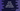# Kotlin for loop explanation with examples# Iterate using a for loop in Kotlin :

for loop in Kotlin is used to iterate through an iterator. You can iterate through array, map or anything that provides an iterator. In this tutorial, I will show you how to use a for loop in Kotlin with different examples. It’s syntax is :

``````for item in collection{

}``````

Let me show you a couple of different for loop examples :

### Iterate through a range of numbers :

We can use Kotlin range expression to iterate through a range of numbers :

``````fun main() {
for (i in 1..5) println("current value \$i")
}``````

It will print :

``````current value 1
current value 2
current value 3
current value 4
current value 5``````

### Iterate through a range of numbers in reverse order :

Use downTo instead of .. for reverse order :

``````fun main() {
for (i in 5 downTo 1) println("current value \$i")
}``````

It will print :

``````current value 5
current value 4
current value 3
current value 2
current value 1``````

### Iterate through a range of numbers with a step :

You can iterate through a range of numbers with a step :

``````fun main() {
for (i in 5 downTo 1 step 2) println("current value \$i")
}``````

It will print :

``````current value 5
current value 3
current value 1``````

You can also use step with .. operator.

### Iterate through a range of numbers excluding the end element :

We can use the until operator to iterate through a range of numbers without including the last element :

``````fun main() {
for (i in 1 until 4) println("current value \$i")
}``````

It will print :

``````current value 1
current value 2
current value 3``````

Note that until doesn’t work for decreasing order.

### Iterate through the array elements :

Iterating through array elements is easy :

``````fun main() {
var numbers = arrayOf(1, 2, 3, 4, 5)
for (i in numbers) println("Current value \$i")
}``````

It will print :

``````Current value 1
Current value 2
Current value 3
Current value 4
Current value 5``````

### Iterate through the array indices :

Kotlin array has one property called indices that provide index of all values in an array. The index starts at 0. We can also use one for..in loop to iterate through these indices :

``````fun main() {
var numbers = arrayOf(1, 2, 3, 4, 5)
for (i in numbers.indices) println("Current value \$i")
}``````

Output :

``````Current value 0
Current value 1
Current value 2
Current value 3
Current value 4``````

The index is starting from 0.

You can also use the withIndex() method of Kotlin array to read both index and value simultaneously :

``````fun main() {
var numbers = arrayOf("one", "two", "three", "four", "five")
for ((index,value) in numbers.withIndex()) println("\$index : \$value")
}``````

### Iterate through a string :

We can iterate through a string using a for..in loop :

``````fun main() {
var str = "hello"
for (c in str) println("current character \$c")
}``````

It will print :

``````current character h
current character e
current character l
current character l
current character o``````

You can also use str.indices to get the indices of a string and iterate through the characters using index :